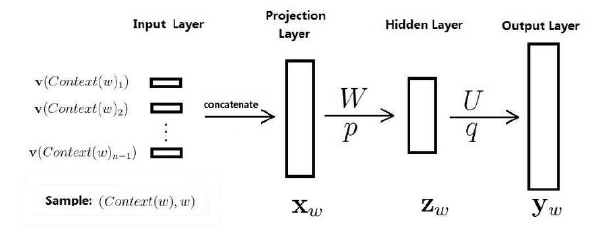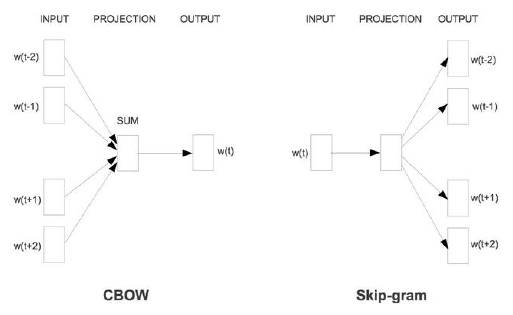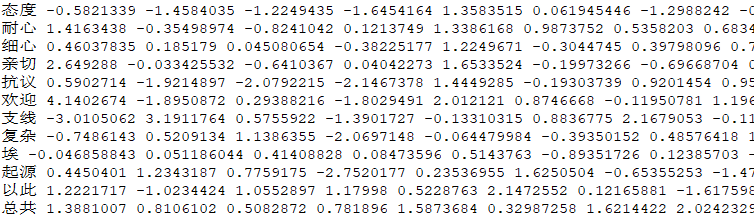## 【NLP】之 Word2vec(将评论转为词向量)_将评论数据转化为向量模型_牧心.的博客-程序员秘密

### 1.Word2vec简介

Word2vec，为一些用来产生词向量的有关模型。这些模型是浅层的神经网络，经过训练可以重新建立语言文本。网络用文字表示，有必要猜测相邻位置的输入字。图1 神经网络模型

Word2vec的实质是用来计算词向量的CBOW模型和Skip-gram模型。

CBOW是一种根据上下文的词语预测当前词语的出现概率的模型。训练CBOW模型的输入数据是某个特征词的上下文相关的词相对应的词向量，其输出数据是这个特定词的词向量。在训练CBOW模型时，词向量只是个副产品，换句话说，词向量是CBOW模型的一个参数。训练开始的时候，词向量初始化为一个随机值，在模型训练的过程中，词向量进行不断地更新。投影层对其求和，也就是简单的向量加法。对于输出层，由于语料库中的词汇量是固定的C个，所以可以将模型的训练看作是多分类问题：对于给定特征，模型从C个分类中输出一个最近的。CBOW模型中上下文距离是可以自定义的，使用的是词袋模型，所以上下文中的词都是平等的，不需考虑其与关注词的距离。

Skip-Gram模型和CBOW模型正好相反，其输入数据是一个特定词的词向量，输出结果是输入的词对应的上下文词向量。Skip-Gram模型的实质是计算输入的词的输入向量  和目标词的输出向量之间的余弦相似度，并进行softmax归一化。

CBOW模型和Skip-Gram模型的原理图如图2。图2 CBOW模型和Skip-Gram模型的原理图

### 2.Word2vec训练

Word2vec在使用前需要线进行训练，本文训练使用的语料库是Wiki百科的中文语料库（1.61G，337336篇文章）。

（1）数据抽取。

``````import logging
import os.path
import sys
import warnings
warnings.filterwarnings(action='ignore', category=UserWarning, module='gensim')
from gensim.corpora import WikiCorpus

if __name__ == '__main__':
program = os.path.basename(sys.argv)  # 得到文件名
logger = logging.getLogger(program)

logging.basicConfig(format='%(asctime)s: %(levelname)s: %(message)s')
logging.root.setLevel(level=logging.INFO)
logger.info("running %s" % ' '.join(sys.argv))

if len(sys.argv) < 3:
print(globals()['__doc__'] % locals())
sys.exit(1)
inp, outp = sys.argv[1:3]
space = " "
i = 0
output = open("out.txt", "w", encoding='utf-8')
# gensim里的维基百科处理类WikiCorpus
wiki = WikiCorpus(inp, lemmatize=False, dictionary=[])
# 通过get_texts将维基里的每篇文章转换位1行text文本
# 并且去掉了标点符号等内容
for text in wiki.get_texts():
output.write(space.join(text) + "\n")
i = i + 1
if (i % 1000 == 0):
logger.info("Saved " + str(i) + " articles.")
output.close()
logger.info("Finished Saved " + str(i) + " articles.")``````

（2）数据预处理。

``opencc -i wiki_zh_1.6g.txt -o wiki_zh_simp.txt -c t2s.json``

``````import logging
import os.path
import sys
import multiprocessing
from gensim.corpora import WikiCorpus
from gensim.models import Word2Vec
from gensim.models.word2vec import LineSentence

if __name__ == '__main__':
program = os.path.basename(sys.argv)
logger = logging.getLogger(program)
logging.basicConfig(format='%(asctime)s: %(levelname)s: %(message)s')
logging.root.setLevel(level=logging.INFO)
logger.info("running %s" % ' '.join(sys.argv))
# check and process input arguments
if len(sys.argv) < 4:
print (globals()['__doc__'] % locals())
sys.exit(1)
inp, outp1, outp2 = sys.argv[1:4]
model = Word2Vec(LineSentence(inp), size=200, window=5, min_count=5, workers=2)
model.save(outp1)
model.wv.save_word2vec_format(outp2, binary=False)``````

``python word2vec_model.py out.txt out.model out.vector``

### 3.用Word2vec将评论转为词向量

``````from gensim.models import Word2Vec
import codecs, sys
import pymysql
import numpy
numpy.set_printoptions(suppress=True)

fcoms = codecs.open('coms_all.txt', 'r', encoding="utf8")
w = codecs.open('vec_all.txt', 'w', encoding="utf8")
size = 200

def count_vec_sentence():
i = 0
id = 0
while line :
words = line.split(' ')
vec = numpy.zeros(size).reshape((1, size))
vec0 = numpy.zeros(size).reshape((1, size))
count = 0
flag = True
for item in words :
word = item.strip()
if word.__len__() > 0 :
if flag :
attitude = word
flag = False
else :
try :
vec += w2v_model[word].reshape((1, size))
count += 1
except KeyError :
# print('==== fault: ', word)
continue
if i % 100 == 0 :
print(i)
i += 1
if count != 0 :
vec /= count
w.write(attitude + ' ')
w.write(str(vec)[2:-2])
w.write('\$')

if '__main__' == __name__:
count_vec_sentence()``````### C# 经典排序算法大全_c# point 排序_NetSuite - 张老师的博客-程序员秘密

C# 经典排序算法大全选择排序using System;using System.Collections.Generic;using System.Linq;using System.Text;namespace sorter{ public class SelectionSorter { private int min; pub

### 雷果国：从1.5K到18K 一个程序员的5年成长之路_csu_小王子的博客-程序员秘密

CSDN雷果国专访：http://www.csdn.net/article/2013-05-13/2815252雷果国《从1.5K到18K，一个程序员的5年成长之路》原文：http://blog.csdn.net/lgg201/article/details/8637763

PGL课程图神经网络学习

### kali 2.0 默认mysql_kali 2.0下搭建DVWA环境_蚕月拾肆的博客-程序员秘密

DVWA (Dam Vulnerable Web Application)DVWA是用PHP+Mysql编写的一套用于常规WEB漏洞教学和检测的WEB脆弱性测试程序。包含了SQL注入、XSS、盲注等常见的一些安全漏洞，在kali linux下搭建DVWA非常方便，因为所需的apache2、mysql、php等环境在kali linux中默认已经安装好了搭建步骤:1. 首先去github上下载DVW...